• Call Now

1800-102-2727•

# Centroid: Definition, Formula, Properties, Practice Problems and FAQs

In a triangle, there are many centres, unlike a circle which has only one centre. Some of the most common centres of a triangle are centroid, incentre,ex-centre, circumcentre and orthocentre. In this article, we are going to learn about the centroid of a triangle in detail. The centroid, also known as the geometrical centre is basically the point of intersection of the medians i.e the line joining the mid-point of sides and the opposite vertices.

• Definition of centroid
• Properties of Centroid
• Centroid Formula
• Practice Problems
• FAQs

## Definition of centroid

The centroid of a triangle is defined as a point of concurrency of the medians i.e, lines joining the mid-point of sides and the opposite vertices of a triangle. It is generally denoted by G. Referring to the figure above, ABC is a triangle AD, BE and CF are the medians of the triangle which intersect at a common point ‘G’ known as the centroid of the triangle ABC . Here points D, E, and F are the mid-points of the side BC, CA and AB respectively.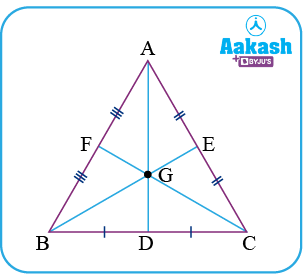## Properties of Centroid

Following are the important properties of the centroid of a triangle -

• It is located at the point of intersection of the medians
• Centroid always divides the median internally in the ratio of 2:1 from the vertex.
• Centroid divides the triangle into three parts of equal areas
• The centroid of a triangle always lies inside the triangle

## Centroid Formula

Given the coordinates of a triangle as A(x1,y1), B(x2,y2)and C(x3,y3), then the coordinates of the centroid is given by-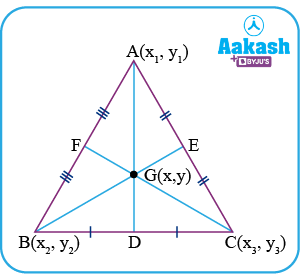$=\frac{{\sum }_{i=1}^{3}{x}_{i}}{3},\frac{{\sum }_{i=1}^{3}{y}_{i}}{3}$

## Derivation for finding the centroid of a triangle (Proof)

Let ABC be a triangle having coordinates A(x1,y1), B(x2,y2) and C(x3,y3) and G(x,y) be the centroid of the triangle.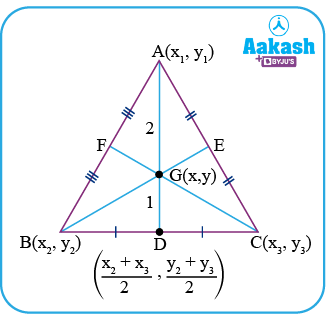Since, AD, BE and CF are the medians of the triangle then D, E and F become the mid-point of the side BC, AC and AB respectively.

As D is the mid-point of BC then using mid-point formula co-ordinates of D is given by -From the properties of centroid, we know that G divides the median in the ratio of 2:1

Therefore, on applying section formula on the median AD we have,Therefore, co-ordinates of the centroid (G) is given by## Practice Problems

1. If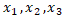are the roots of the equation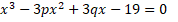then, find the centroid of Δ ABC  having coordinates as A(x 1, 1/ x 1), B(x2 ,1/ x2 ),C( x3 , 1/ x3 ) as shown in the figure given below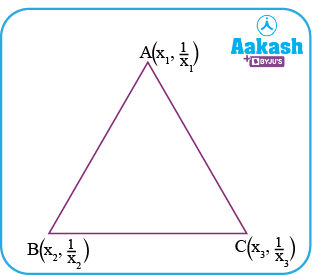Solution-   Given,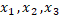are the roots of the equationthen ,

Sum of roots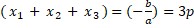Sum of product of roots taken two at a time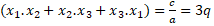Product of roots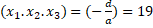We know that, centroid of a triangle G(x,y) is given by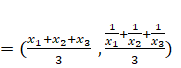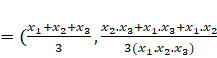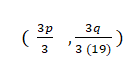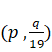Therefore coordinates of centroid are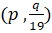1. In a ABC, coordinates of B = (0,0) , AB= 2 units , ∠ABC = 600 and mid-point of BC = (2,0) , then find the centroid of ABC.
Solution - Let D be the mid-point of BC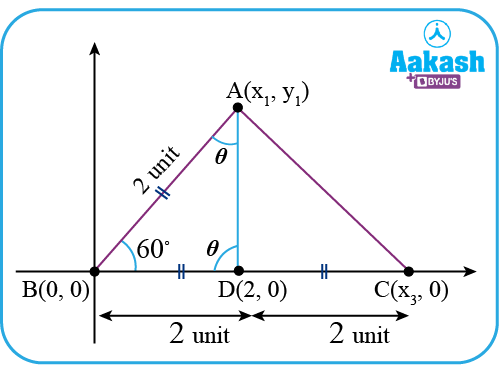∴ coordinates of D=(2,0)

Hence, coordinates of C(x3,0) becomes (4,0)[∵BD = DC]

Now, in Δ ABD, we have
AB=BD= 2 units

∴ ∠ADB = ∠BAD = θ (say) [Angles opposite to equal sides are equal]

Also, in Δ ABD

60+ = θ  + θ = 1800     [Angle sum property of a triangle]

θ = 600

Therefore, Δ ABD becomes an equilateral triangle

Now, applying distance formula between A and B, we have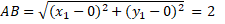Squaring both sides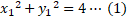Again applying distance formula between A and D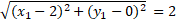Squaring both sides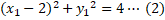Solving equation (1) and (2)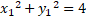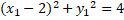___________________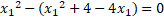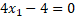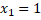Putting = 1 in equation (1)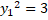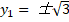Therefore, coordinates of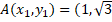)

Note-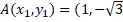) is neglected because in the problem ABC is given as 600 so , the coordinate of A is going to lie in the first quadrant.

Now, the centroid of  Δ ABC i.e, G(x,y) =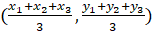=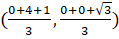=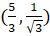1. Find the centroid of the triangle made by the lines x + y = 2 and x y = 0 .

Solution: Given
x . y = 0
∴ either x = 0 or y = 0

Therefore, the lines are x = 0 , y = 0 and x + y = 2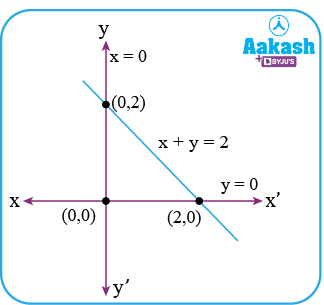The centroid of the triangle G(x,y) =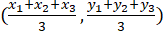=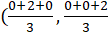=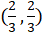1. If (1, 1) are the co-ordinates of the centroid of a triangle whose vertices are (0, 4), (-2,0) and (p, q). Determine the value of p,q

Solution:

Given (x1,y1)=(0,4)
(x2,y2) = (-2,0)
(x3,y3) = (p,q)

We know that, Centroid of a triangle is given by G(x,y) =Hence,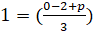and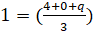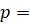5                                q= -1

## FAQs

Ques 1) If ‘P is any internal point of a triangle such that area of △APB, △BPC and △APC are equal then P must be a ………………..
As ‘P’ is an internal point of the triangle and it is dividing the triangle into three parts of equal area then P must be the centroid of the triangle.

Ques 2) What will be the location of the centroid in a right isosceles triangle? (inside/outside/on the hypotenuse/ at right-angled vertex)
The position of a centroid is always inside the triangle as it is made by the intersection point of medians.

Ques 3) What is the relation between orthocentre(H), centroid(G) and circumcentre(O)?
Orthocentre, centroid and circumcentre are always collinear and the centroid divides the line joining it in the ratio of 2:1 internally (except in an equilateral triangle)Ques 4) What is the relation between orthocentre, centroid and circumcentre in the case of an equilateral triangle?
In the case of an equilateral triangle, all three points centroid, circumcentre and orthocentre lie on the same point or coincide with each other.Talk to our expert
Resend OTP Timer =
By submitting up, I agree to receive all the Whatsapp communication on my registered number and Aakash terms and conditions and privacy policy